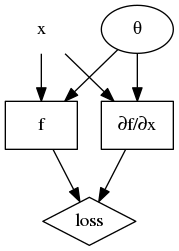# How to understand autograd.grad function

Hi,

The grad function can be used to calculate partial derivative according to this post. As per documentation,

If `create_graph=True` , graph of the derivative will be constructed, allowing to compute higher order derivative products.

and `retain_graph` will be automatically set to `True`. However, I’m a bit confused how it works, or in other words, what should the computation graph look like by using this option.

As a tractable example, let x be a known vector, f be a `torch.nn.Module` instance that returns a scalar, and θ be the parameters of f:To implement this loss, which will be subsequently differentiated against θ, I think a good implementation should:

• not compute gradient with respect to θ when evaluating the partial gradient with respect to x
• have the two occurrences of x sharing the same data
• contain only one graph for f(x;θ)
• treat the partial derivative in the second term as a new function with respect to θ

The computation graph I’d like to achieve should appear as: (legend: node without border -> requires_grad=False; node in oval border -> requires_grad=True; node in rectangle border -> `torch.nn.Module` instance or similar entity in a computation graph; node in diamond border -> the scalar output i.e. the loss)To attain the desired computation graph I may write the following code (version: `PyTorch-0.4.1`):

``````# `x` is the input such that x.requires_grad is False;
# `f` is the network
# As a specific example, assume that:
# x = torch.rand(3)
# f = torch.nn.Linear(3, 1)
import torch
from torch.autograd import grad
# build graph for f(x;theta) without derivative
loss1 = f(x)
# only_inputs=True: so that gradient of f(x) with respect to theta is not evaluated here
# create_graph=True: so that `dfdx` can be used as intermediate node in other graph
# retain_graph=False: so that we won't have a duplicate graph for f(x)
x_ = x.detach().requires_grad_()
dfdx, = grad(f(x_), x_, only_inputs=True, create_graph=True, retain_graph=False)
loss2 = torch.norm(dfdx, 1)
loss = loss1 + loss2
loss.backward()
``````

This code snippet certainly compiles. However, it’s quite difficult for me to directly verify the result is correct. My questions here are:

• How to visualize the computation graph created by `create_graph=True` in the above simple code?
• Does `retain_graph=False` do what I intend to do as shown in comment? What if I change to `retain_graph=True`?
• Is there a way to not compute `f(x)` twice while maintaining the same computation graph?

Thanks for reading till here! Thanks in advance for your help!

Hi,

I am coding on the similar los function. Did you finally figure out if this is correct? Thank you very much.